# Absolute value

(diff) ← Older revision | Latest revision (diff) | Newer revision → (diff)

modulus, of a real number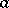The non-negative number, denoted by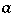, which is defined as follows: If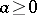,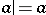; if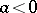,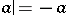. The absolute value (modulus) of a complex number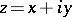, whereandare real numbers, is the number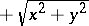. Absolute values obey the following relations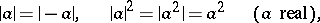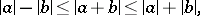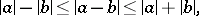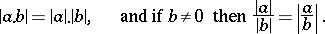A generalization of the concept of the absolute value to the case of arbitrary fields exists, cf. Norm on a field.

How to Cite This Entry:
Absolute value. Encyclopedia of Mathematics. URL: http://encyclopediaofmath.org/index.php?title=Absolute_value&oldid=28824